# cusumtest

Cusum test for structural change

## Syntax

``h = cusumtest(X,y)``
``h = cusumtest(Tbl)``
``h = cusumtest(___,Name=Value)``
``````[h,H,Stat,W,B] = cusumtest(___)``````
``cusumtest(___)``
``cusumtest(ax,___)``
``````[___,sumPlots] = cusumtest(___)``````

## Description

Cusum tests assess the stability of coefficients β in a multiple linear regression model of the form y = + ε. Inference is based on a sequence of sums, or sums of squares, of recursive residuals (standardized one-step-ahead forecast errors) computed iteratively from nested subsamples of the data. Under the null hypothesis of coefficient constancy, values of the sequence outside an expected range suggest structural change in the model over time.

example

````h = cusumtest(X,y)` returns test rejection decision `h` from conducting a cusum test on the multiple linear regression model y = Xβ + ε, where `y` is a vector of response data and `X` is a matrix of predictor data.```

example

````h = cusumtest(Tbl)` conducts a cusum test on the variables of the table or timetable `Tbl`. The response variable in the regression is the last table variable, and all other variables are the predictor variables. To select a different response variable for the regression, use the `ResponseVariable` name-value argument. To select different predictor variables, use the `PredictorNames` name-value argument.```

example

````h = cusumtest(___,Name=Value)` uses additional options specified by one or more name-value arguments. Some options control the number of tests to conduct. The following conditions apply when `cusumtest` conducts multiple tests: `cusumtest` treats each test as separate from all other tests.All outputs expand their singleton dimension to contain results from each test. For example, ```cusumtest(Tbl,ResponseVariable="RGDP",Test=["cusum" cusumsq"])``` conducts two cusum tests using `GDP` as the response variable in the regressions and all other variables in the table `Tbl` as predictors. The first test uses the cusum test statistic and the second test uses the cusum of squares test statistic.```

example

``````[h,H,Stat,W,B] = cusumtest(___)``` also returns the following decision statistics from conducting a cusum test, using any input-argument combination in the previous syntaxes: `h`, the test decision`H`, the sequence of decisions for each iteration of the test`Stat`, the sequence of test statistics`W`, the sequence of recursive residuals`B`, the sequence of coefficient estimates ```

example

````cusumtest(___)` plots both the sequence of cusums and the critical lines resulting from the cusum tests.```
````cusumtest(ax,___)` plots on the axes specified by `ax` instead of the current axes (`gca`). `ax` can precede any of the input argument combinations in the previous syntaxes.```
``````[___,sumPlots] = cusumtest(___)``` additionally returns handles to plotted graphics objects. Use elements of `sumPlots` to modify properties of the plot after you create it.```

## Examples

collapse all

Conduct a cusum test to assess whether there is a structural break in the equation for food demand. Input the predictor series as a matrix and input the response series as a vector.

Load the US food consumption data set `Data_Consumption.mat`, which contains annual measurements from 1927 through 1962 with missing data due to World War II in the matrix `Data`.

`load Data_Consumption`

Suppose that you want to develop a model for consumption as determined by food prices and disposable income, and assess its stability through the economic shock through the war.

Plot the series.

```P = Data(:,1); % Food price index I = Data(:,2); % Disposable income index Q = Data(:,3); % Food consumption index figure; plot(dates,[P I Q]) axis tight grid on xlabel("Year") ylabel("Index") legend(["Price" "Income" "Consumption"],Location="southeast")```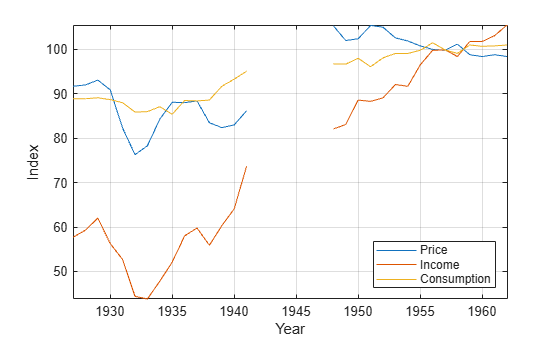Measurements are missing from 1942 through 1947, which correspond to WWII.

Stabilize each series by applying the log transformation.

```LP = log(P); LI = log(I); LQ = log(Q);```

Assume that log consumption is a linear function of the logs of food price and income.

`${\text{LQ}}_{t}={\beta }_{0}+{\beta }_{1}{\text{LI}}_{t}+{\beta }_{2}\text{LP}+{\epsilon }_{t}.$`

${\epsilon }_{t}$ is a Gaussian random variable with mean 0 and standard deviation ${\sigma }^{2}$.

Identify the indices before WWII. Plot log consumption with respect to the logs of food price and income.

```preWarIdx = (dates <= 1941); figure scatter3(LP(preWarIdx),LI(preWarIdx),LQ(preWarIdx),[],"ro"); hold on scatter3(LP(~preWarIdx),LI(~preWarIdx),LQ(~preWarIdx),[],"b*"); legend(["Pre-war observations" "Post-war observations"], ... Location="best") xlabel("Log Price") ylabel("Log Income") zlabel("Log Consumption") % Obtain better view h = gca; h.CameraPosition = [4.3 -12.2 5.3];```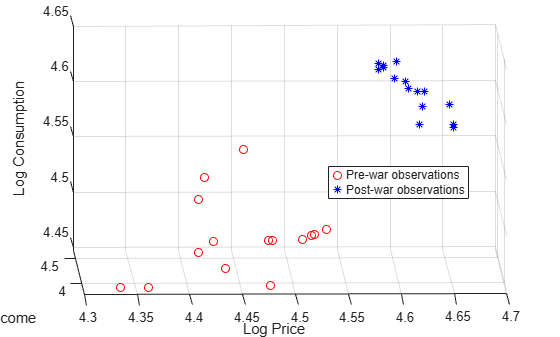Data relationships appear to be affected by the war.

Conduct a cusum test to assess whether there is a significant structural change. Use default values.

```X = [LP LI]; y = LQ; h = cusumtest(X,y)```
```h = logical 0 ```

`h = 0` indicates that there is not enough evidence to reject the null hypothesis that the coefficients are equal across subsamples.

Conduct a cusum test to assess whether there is a structural change in the equation for food demand, where the time series are variables in a table.

Load the US food consumption data set `Data_Consumption.mat`, which contains annual measurements from 1927 through 1962 with missing data due to World War II in the table `DataTable`. Convert the table to a timetable, and remove rows containing missing values.

```load Data_Consumption dates = datetime(dates,12,31); TT = table2timetable(DataTable,RowTimes=dates); TT.Row = []; TT = rmmissing(TT);```

Apply the log transform to all variables in the table.

```LogTT = varfun(@log,TT); LogTT.Properties.VariableNames```
```ans = 1x3 cell {'log_P'} {'log_I'} {'log_Q'} ```

Conduct a cusum test to assess whether there is a structural change in the regression model of log food consumption `log_Q` on log price `log_P` and log income `log_I`.

`h = cusumtest(LogTT)`
```h = logical 0 ```

By default, `chowtest` selects the last table variable as the response, and selects all other variables as predictors. You can select a different variable by using the `ResponseVariable` name-value argument, and you can choose a different set of predictor variables by using the `PredictorVariables` name-value argument.

Load the US food consumption data set `Data_Consumption.mat`. Consider a model for log food consumption as determined by log food prices and log disposable income.

```load Data_Consumption LogDT = varfun(@log,DataTable); numObs = height(LogDT) - sum(any(ismissing(LogDT),2))```
```numObs = 30 ```
`numPreds = width(LogDT) - 1`
```numPreds = 2 ```

Conduct a cusum test using default values. Return all test decision statistics.

`[h,H,Stat,W] = cusumtest(LogDT)`
```h = logical 0 ```
```H=1×28 table H1 H2 H3 H4 H5 H6 H7 H8 H9 H10 H11 H12 H13 H14 H15 H16 H17 H18 H19 H20 H21 H22 H23 H24 H25 H26 H27 H28 _____ _____ _____ _____ _____ _____ _____ _____ _____ _____ _____ _____ _____ _____ _____ _____ _____ _____ _____ _____ _____ _____ _____ _____ _____ _____ _____ _____ Test 1 false false false false false false false false false false false false false false false false false false false false false false false false false false false false ```
```Stat=1×28 table Stat1 Stat2 Stat3 Stat4 Stat5 Stat6 Stat7 Stat8 Stat9 Stat10 Stat11 Stat12 Stat13 Stat14 Stat15 Stat16 Stat17 Stat18 Stat19 Stat20 Stat21 Stat22 Stat23 Stat24 Stat25 Stat26 Stat27 Stat28 _____ _________ ________ ________ ________ ________ _______ _______ _______ _______ ______ ________ ________ ______ ______ ______ ______ ______ ______ ______ ______ ______ ______ ______ ______ _______ ________ ________ Test 1 NaN -0.012438 0.064511 -0.50784 -0.55747 -0.42687 -2.7881 -3.0973 -3.7625 -3.5417 -1.913 -0.65794 -0.35743 2.3762 3.3104 3.7509 2.8851 3.7395 4.2295 4.641 4.2412 4.496 3.2467 2.0001 1.5324 0.81729 0.053352 -0.98812 ```
```W=1×28 table W1 W2 W3 W4 W5 W6 W7 W8 W9 W10 W11 W12 W13 W14 W15 W16 W17 W18 W19 W20 W21 W22 W23 W24 W25 W26 W27 W28 ___ ___________ __________ __________ ___________ _________ _________ __________ __________ _________ ________ ________ ________ ________ _________ _________ __________ _________ _________ _________ __________ _________ _________ _________ __________ __________ __________ _________ Test 1 NaN -0.00012823 0.00079327 -0.0059004 -0.00051169 0.0013464 -0.024342 -0.0031882 -0.0068572 0.0022762 0.016791 0.012938 0.003098 0.028181 0.0096305 0.0045417 -0.0089262 0.0088086 0.0050515 0.0042415 -0.0041209 0.0026269 -0.012879 -0.012851 -0.0048219 -0.0073721 -0.0078755 -0.010737 ```

`cusumtest` returns the overall rejection decision `h`, and tables containing the sequence of rejection decision from the forward recursions of the cusum test `H`, the corresponding sequence of test statistics `Stat`, and the corresponding recursive residuals `W`. Each table contains `numObs - numPreds + Intercept = 28` variables corresponding to results of each recursion in the cusum test. `Stat1 = W1 = NaN` indicates the presence of a model intercept.

Determine whether an explanatory model of real gross national product (RGNP) is stable by plotting recursive residuals.

Load the Nelson-Plosser data set `Data_NelsonPlosser.mat`, which contains the table of data `DataTable`.

`load Data_NelsonPlosser`

The time series in the data set contain annual, macroeconomic measurements from 1860 to 1970. For more details, a list of variables, and descriptions, enter `Description` in the command line.

Convert the table to a timetable. Focus the sample to measurements from the end of 1915 through the end of 1970.

```dates = datetime(dates,12,31); span = isbetween(dates,datetime(1915,12,31),datetime(1970,12,31),"closed"); TT = table2timetable(DataTable,RowTimes=dates); TT.Dates = []; TT = TT(span,:);```

Consider a predictive model of the US RGNP `GNPR` given measurements of the industrial production index `IPI`, total employment `E`, and real wages `WR`.

Plot the series in the model.

```prednames = ["IPI" "E" "WR"]; tiledlayout(2,2) for j = ["GNPR" prednames] nexttile plot(TT.Time,TT{:,j}) ylabel(j) end```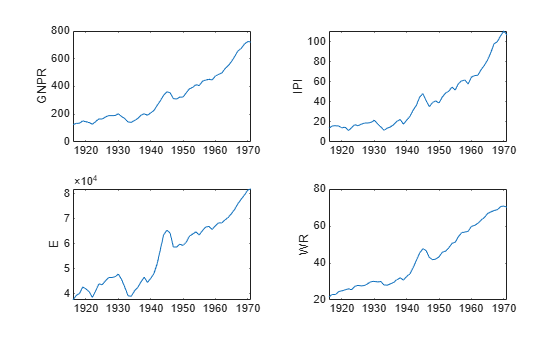To address exponential growth, apply the log transform to the series.

`LogTT = varfun(@log,TT);`

`LogTT` is a timetable containing the transformed variables in `TT`, but with names prepended with `log_`.

Assume that an appropriate multiple regression model to describe real GNP is

`$\mathrm{log}\left({\text{GNPR}}_{t}\right)={\beta }_{0}+{\beta }_{1}\mathrm{log}\left({\text{IPI}}_{t}\right)+{\beta }_{2}\mathrm{log}\left({\text{E}}_{t}\right)+{\beta }_{3}\mathrm{log}\left({\text{WR}}_{t}\right).$`

Conduct a cusum test to assess whether all regression coefficients are stable. Print a test summary to the command line. Plot the test statistics.

```lprednames = "log_" + prednames; cusumtest(LogTT,ResponseVariable="log_GNPR", ... PredictorVariables=lprednames,Display="summary")```
```RESULTS SUMMARY *************** Test 1 Test type: cusum Test direction: forward Intercept: yes Number of iterations: 52 Decision: Fail to reject coefficient stability Significance level: 0.0500 ```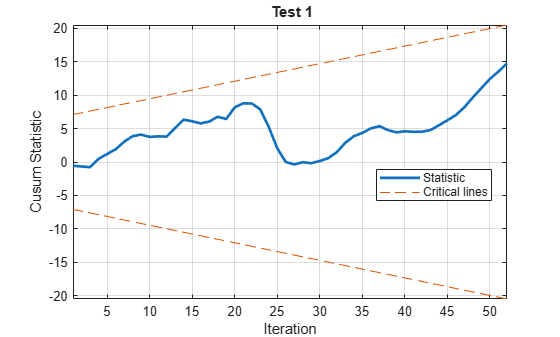The cusum series does not cross the critical lines, which indicates model stability.

Conduct cusum tests to assess whether there are structural changes in the equation for food demand around World War II. Implement forward and backward recursive regressions to obtain the test statistics.

Load the US food consumption data set `Data_Consumption.mat`, which contains annual measurements from 1927 through 1962 with missing data due to the World War II in the table `DataTable`. Convert the table to a timetable, and remove rows containing missing values.

```load Data_Consumption dates = datetime(dates,12,31); TT = table2timetable(DataTable,RowTimes=dates); TT.Row = []; TT = rmmissing(TT);```

Consider a model for log food consumption as determined by log food prices and log disposable income, and assess its stability through the economic shock through the war.

Apply the log transform to all variables in the table.

`LogTT = varfun(@log,TT);`

Conduct forward and backward cusum tests using a 5% level of significance for each test. Plot the cusums. Return the recursive residuals.

```[h,~,~,W] = cusumtest(LogTT,Direction=["forward" "backward"], ... Plot="on");```
```RESULTS SUMMARY *************** Test 1 Test type: cusum Test direction: forward Intercept: yes Number of iterations: 27 Decision: Fail to reject coefficient stability Significance level: 0.0500 ```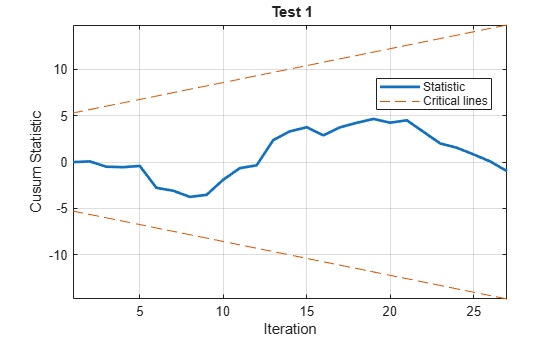```*************** Test 2 Test type: cusum Test direction: backward Intercept: yes Number of iterations: 27 Decision: Fail to reject coefficient stability Significance level: 0.0500 ```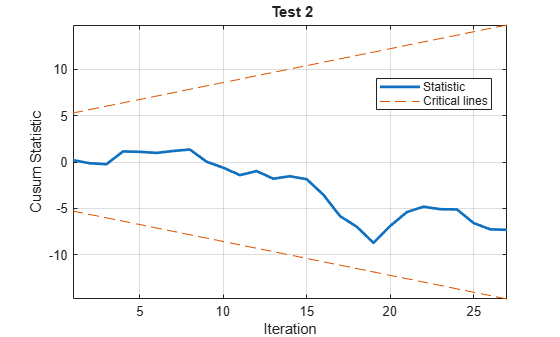The plots and test results at the command line indicate that neither test rejects the null hypothesis that coefficients are stable.

Compare the results of the cusum tests with the results of a Chow test. Unlike cusum tests, Chow tests require a guess for the time point at which the structural break occurs. Specify that the break point is 1941.

```bp = find(LogTT.Time >= datetime(1941,12,31),1); chowtest(LogTT,bp,Display="summary");```
```RESULTS SUMMARY *************** Test 1 Sample size: 30 Breakpoint: 15 Test type: breakpoint Coefficients tested: All Statistic: 5.5400 Critical value: 3.0088 P value: 0.0049 Significance level: 0.0500 Decision: Reject coefficient stability ```

The test results reject the null hypothesis that the coefficients are stable.

The Chow and cusum test results are not consistent. For details on cusum test limitations, see Limitations.

Check whether a cusum of squares test can detect a structural break in volatility in simulated data.

Simulate a series of data from this regression model

`$\left\{\begin{array}{c}{y}_{t}=\left[\begin{array}{ccc}1& 2& 3\end{array}\right]{x}_{t}+{\epsilon }_{1t};\phantom{\rule{0.2777777777777778em}{0ex}}t=1,...,50\\ {y}_{t}=\left[\begin{array}{ccc}1& 2& 3\end{array}\right]{x}_{t}+{\epsilon }_{2t};t=51,...,100.\end{array}$`

${x}_{t}$ is a series of observations from three standard Gaussian predictor variables. ${\epsilon }_{1t}$ and ${\epsilon }_{2t}$ are series of Gaussian innovations both with mean 0 and standard deviation 0.1 and 0.2, respectively.

```rng(1); % For reproducibility T = 100; X = randn(T,3); sigma1 = 0.1; sigma2 = 0.2; e = [sigma1*randn(T/2,1); sigma2*randn(T/2,1)]; b = (1:3)'; y = X*b + e;```

Conduct a cusum of squares test using a 5% level of significance. Plot the test statistics and critical region bands. Indicate that there is no model intercept. Request to return whether the test statistics cross into critical region at each iteration.

```[~,H] = cusumtest(X,y,Test="cusumsq",Plot="on", ... Direction=["forward" "backward"],Display="off", ... Intercept=false);```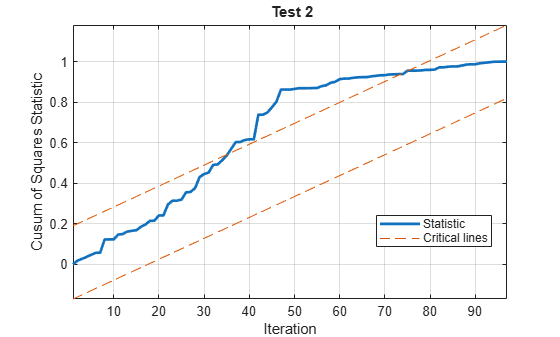Because the test statistics cross the critical lines at least once for both tests, the tests reject the null hypothesis of constant volatility at 5% level. The test statistics change direction around iteration 50, which is consistent with the simulated break in volatility in the data.

`H` is a 2-by-97 logical matrix containing the sequence of decisions for each iteration of each cusum of squares test. The first row corresponds to the forward cusum of squares test, and the second row corresponds to the backward cusum of squares test.

For the forward test, determine the iterations that result in the test statistics crossing the critical line.

`bp = find(H(1,:) == 1)`
```bp = 1×35 24 25 26 27 28 29 30 31 32 33 34 35 36 37 38 39 40 41 42 43 44 45 46 47 48 49 50 51 52 53 54 55 56 57 58 ```

## Input Arguments

collapse all

Predictor data X for the multiple linear regression model, specified as a `numObs`-by-`numPreds` numeric matrix.

Each row represents one of the `numObs` observations and each column represents one of the `numPreds` predictor variables.

Data Types: `double`

Response data y for the multiple linear regression model, specified as a `numObs`-by-1 numeric vector. Rows of `y` and `X` correspond.

Data Types: `double`

Combined predictor and response data for the multiple linear regression model, specified as a table or timetable with `numObs` rows. Each row of `Tbl` is an observation.

The test regresses the response variable, which is the last variable in `Tbl`, on the predictor variables, which are all other variables in `Tbl`. To select a different response variable for the regression, use the `ResponseVariable` name-value argument. To select different predictor variables, use the `PredictorNames` name-value argument to select `numPreds` predictors.

Axes on which to plot, specified as a vector of `Axes` objects with length `numTests`.

By default, `cusumtest` plots each test to a separate figure.

Note

`NaN`s in `X`, `y`, or `Tbl` indicate missing values, and `cusumtest` removes observations containing at least one `NaN`. That is, to remove `NaN`s in `X` or `y`, `cusumtest` merges the variables ```[X y]```, and then it uses list-wise deletion to remove any row that contains at least one `NaN`. `cusumtest` also removes any row of `Tbl` containing at least one `NaN`. Removing `NaN`s in the data reduces the sample size and can create irregular time series.

### Name-Value Arguments

Specify optional pairs of arguments as `Name1=Value1,...,NameN=ValueN`, where `Name` is the argument name and `Value` is the corresponding value. Name-value arguments must appear after other arguments, but the order of the pairs does not matter.

Before R2021a, use commas to separate each name and value, and enclose `Name` in quotes.

Example: ```cusumtest(Tbl,ResponseVariable="RGDP",Test=["cusum" cusumsq"])``` conducts two cusum tests using `GDP` as the response variable in the regressions and all other variables in the table `Tbl` as predictors. The first test uses the cusum test statistic and the second test uses the cusum of squares test statistic.

Flag to include an intercept when `cusumtest` fits the regression model, specified as a value in this table or a length `numTests` vector of such values.

ValueDescription
`true``cusumtest` includes an intercept when fitting the regression model. `numCoeffs` = `numPreds` + 1.
`false``cusumtest` does not include an intercept when fitting the regression model. `numCoeffs` = `numPreds`.

`cusumtest` conducts a separate test for each value in `Intercept`.

Example: `Intercept=false` excludes an intercept from the model for each test.

Data Types: `logical`

Type of cusum test, specified as a test name, or a string vector or cell vector of test names of length `numTests`.

Test NameDescription
`"cusum"`Cusum test statistic. See .
`"cusumsq"`Cusum of squares test statistic. See .

`cusumtest` conducts a separate test for each test name in `Test`.

Example: `Test=["cusum" "cusumsq"]` conducts two cusum tests. The first test uses the cusum test statistic and the second test uses the cusum of squares test statistic.

Data Types: `char` | `cell` | `string`

Iteration direction, specified as a direction name, or a string vector or cell vector of direction names of length `numTests`.

Direction NameDescription
`"forward"`

`cusumtest` computes recursive residuals beginning with the first `numCoeffs` + 1 observations. Then, `cusumtest` adds one at a time until it reaches `numObs` observations.

`"backward"`

`cusumtest` reverses the order of the observations, and then follows the same steps as in `"forward"`.

`cusumtest` conducts a separate test for each value in `Direction`.

Example: `Test=["cusum" "cusumsq"]` conducts two cusum tests. The first test computes recursive residuals using the forward method and the second test computes recursive residuals using the backward method.

Data Types: `char` | `cell` | `string`

Nominal significance levels for the tests, specified as a numeric scalar or numeric vector of length `numTests`.

• For cusum tests (`Test="cusum"`), all elements of `Alpha` must be in the interval (0,1).

• For cusum of squares tests (`Test="cusumsq"`), all elements of `Alpha` must be in the interval [0.01,0.20].

`cusumtest` conducts a separate test for each value in `Alpha`.

Example: `Alpha=[0.01 0.05]` uses a level of significance of `0.01` for the first test, and then uses a level of significance of `0.05` for the second test.

Data Types: `double`

Flag for a command window display of results, specified as a value in this table.

ValueDescriptionDefault Value When
`"off"``cusumtest` does not display results in the command window.`numTests` = 1
`"summary"`For each test, `cusumtest` displays results in the command window.`numTests` > 1

The value of `Display` applies to all tests.

Example: `Display="off"`

Data Types: `char` | `string`

Flag indicating whether to plot test results, specified as a value in this table.

ValueDescriptionDefault Value When
`"off"``cusumtest` does not produce any plots.`cusumtest` returns any output argument.
`"on"``cusumtest` produces individual plots for each test.`cusumtest` does not return any output arguments.

Depending on the value of `Test`, the plots show the sequence of cusums or cusums of squares together with critical lines determined by the value of `Alpha`.

The value of `Plot` applies to all tests.

Example: `Plot="off"`

Data Types: `char` | `string`

Variable in `Tbl` to use for response, specified as a string vector or cell vector of character vectors containing variable names in `Tbl.Properties.VariableNames`, or an integer or logical vector representing the indices of names. The selected variables must be numeric.

`cusumtest` uses the same specified response variable for all tests.

Example: `ResponseVariable="GDP"`

Example: `ResponseVariable=[true false false false]` or `ResponseVariable=1` selects the first table variable as the response.

Data Types: `double` | `logical` | `char` | `cell` | `string`

Variables in `Tbl` to use for the predictors, specified as a string vector or cell vector of character vectors containing variable names in `Tbl.Properties.VariableNames`, or an integer or logical vector representing the indices of names. The selected variables must be numeric.

`cusumtest` uses the same specified predictors for all tests.

By default, `cusumtest` uses all variables in `Tbl` that are not specified by the `ResponseVariable` name-value argument.

Example: `PredictorVariables=["UN" "CPI"]`

Example: `PredictorVariables=[false true true false]` or `DataVariables=[2 3]` selects the second and third table variables.

Data Types: `double` | `logical` | `char` | `cell` | `string`

Note

• When `cusumtest` conducts multiple tests, the function applies all single settings (scalars or character vectors) to each test.

• All vector-valued specifications that control the number of tests must have equal length.

• If the value of any option is a row vector, so is output `h`. Array and table outputs retain their specified dimensions.

## Output Arguments

collapse all

Test rejection decisions, returned as a logical scalar or vector with length equal to the number of tests `numTests`. `cusumtest` returns `h` when you supply the inputs `X` and `y`.

Hypotheses are independent of the value of `Test`.

• H0: Coefficients in β are equal in all sequential subsamples.

• H1: Coefficients in β change during the period of the sample.

Elements of `h` have the following values and meanings.

• Values of `1` indicates rejection of H0 in favor of H1.

• Value of `0` indicates failure to reject H0.

Sequence of test rejection decisions for each iteration of the cusum tests, returned as a `numTests`-by-(`numObs``numPreds`) logical matrix or table of logical variables.

Rows correspond to separate cusum tests and columns or variables correspond to iterations. When `H` is a table, variable `j` has label `Hj`.

• For tests in which `Direction` is `"forward"`, columns or variables correspond to times `numPreds` + 1,...,`numObs`.

• For tests in which `Direction` is `"backward"`, columns or variables correspond to times `numObs` – (`numPreds` + 1),...,1.

Rows corresponding to tests in which `Intercept` is `true` contain one less iteration, and the value in the first column of `H` defaults to `false`.

For a particular test (row), if any test decision in the sequence is `1`, then `h` is `true`; that is, `h = any(H,2)`. Otherwise, `h` is `false`.

Sequence of test statistics for each iteration of the cusum tests, returned as a `numTests`-by-(`numObs``numPreds`) numeric matrix or table of numeric variables.

Rows correspond to separate cusum tests and columns or variables correspond to iterations. When `Stat` is a table, variable `j` has label `Statj`.

Values in any row depend on the value of `Test`. Array indices corresponds to the indexing in `H`. When `W` is a table, variable `j` has label `Wj`.

Rows corresponding to tests in which `Intercept` is `true` contain one less iteration, and the value in the first column of `Stat` defaults to `NaN`.

Sequence of standardized recursive residuals, returned as a `numTests`-by-(`numObs``numPreds`) numeric matrix or table of numeric variables.

Values in any row depend on the value of `Test`. Array indices correspond to the indexing in `H`. When `W` is a table, variable `j` has label `Wj`.

Rows corresponding to tests in which `Intercept` is `true` contain one less iteration, and the value in the first column of `W` defaults to `NaN`.

Sequence of recursive regression coefficient estimates, returned as a (`numPreds` + 1)-by-(`numObs``numPreds`)-by-`numTests` numeric array.

• `B(i,j,k)` corresponds to coefficient `i` at iteration `j` for test `k`.

At iteration `j` of test `k`, `cusumtest` estimates the coefficients using

`B(:,j,k) = X(1:numPreds+j,inRegression)\y(1:numPreds+j);`
`inRegression` is a logical vector indicating the predictors in the regression at iteration `j` of test `k`.

• During forward iterations, initially constant predictors can cause multicollinearity. Therefore, `cusumtest` holds out constant predictors until their data changes. For iterations in which `cusumtest` excludes predictors from the regression, corresponding coefficient estimates default to `NaN`. Similarly, for backward regression, `cusumtest` holds out terminally constant predictors. For more details, see .

• Tests in which:

• `Intercept` is `true` contain one less iteration, and all values in the first column of `B` default to `NaN`.

• `Intercept` is `false` contain one less coefficient, and the value in the first row, which corresponds to the intercept, defaults to `NaN`.

Handles to plotted graphics objects, returned as a `3`-by-`numTests` graphics array. `sumPlots` contains unique plot identifiers, which you can use to query or modify properties of the plot.

## Limitations

Cusum tests have little power to detect structural changes in the following cases.

• Late in the sample period

• When multiple changes produce cancellations in the cusums

collapse all

### Cusum Tests

Cusum tests provide useful diagnostics for various model misspecifications, including gradual structural change, multiple structural changes, missing predictors, and neglected nonlinearities. The tests, formulated in , are based on cumulative sums, or cusums, of residuals resulting from recursive regressions.

## Tips

• The cusum of squares test:

• Is a “useful complement to the cusum test, particularly when the departure from constancy of the [recursive coefficients] is haphazard rather than systematic” 

• Has greater power for cases in which multiple shifts are likely to cancel

• Is often suggested for detecting structural breaks in volatility

• `Alpha` specifies the nominal significance levels for the tests. The actual size of a test depends on various assumptions and approximations that `cusumtest` uses to compute the critical lines. Plots of the recursive residuals are the best indicator of structural change. Brown, et al. suggest that the tests “should be regarded as yardsticks for the interpretation of data rather than leading to hard and fast decisions” .

• To produce basic diagnostic plots of the recursive coefficient estimates having the same scale for test `n`, enter

`plot(B(:,:,n)')`
`recreg` produces similar plots, optionally using robust standard error bands.

## Algorithms

• `cusumtest` handles initially constant predictor data using the method suggested in  . If a predictor's data is constant for the first `numCoeffs` observations and this results in multicollinearity with an intercept or another predictor, then `cusumtest` drops the predictor from regressions and the computation of recursive residuals until its data changes. Similarly, `cusumtest` temporarily holds out terminally constant predictors from backward regressions. Initially constant predictors in backward regressions, or terminally constant predictors in forward regressions, are not held out by `cusumtest` and can lead to rank deficiency in terminal iterations.

• `cusumtest` computes critical lines for inference in essentially different ways for the two test statistics. For cusums, `cusumtest` solves the normal CDF equation in  dynamically for each value of `Alpha`. For the cusums of squares test, `cusumtest` interpolates parameter values from the table in , using the method suggested in . Sample sizes with degrees of freedom less than 4 are below tabulated values, and `cusumtest` cannot compute critical lines. Sample sizes with degrees of freedom greater than 202 are above tabulated values, and `cusumtest` uses the critical value associated with the largest tabulated sample size.

 Brown, R. L., J. Durbin, and J. M. Evans. "Techniques for Testing the Constancy of Regression Relationships Over Time." Journal of the Royal Statistical Society, Series B. Vol. 37, 1975, pp. 149–192.

 Durbin, J. "Tests for Serial Correlation in Regression Analysis Based on the Periodogram of Least Squares Residuals." Biometrika. Vol. 56, 1969, pp. 1–15.